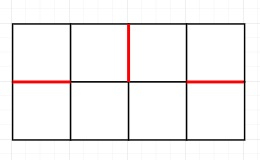# Minimum Cuts can be made in the Chessboard such that it is not divided into 2 parts in Python

PythonServer Side ProgrammingProgramming

Suppose we have one A x B chessboard (matrix), we have to calculate the maximum numbers of cuts that we can make in this board so that the board is not divided into 2 parts.

So, if the input is like A = 2 and B = 4,then the output will be 3

To solve this, we will follow these steps −

• res := 0
• res :=(M - 1) *(N - 1)
• return res

## Example

Let us see the following implementation to get better understanding −

Live Demo

def max_cuts_count(M, N):
res = 0
res = (M - 1) * (N - 1)
return res
M, N = 2, 4
Cuts = max_cuts_count(M, N)
print(Cuts)

## Input:

2,4

## Output

3